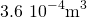1 Units and Measurement

1.3 Unit Conversion

Learning Objectives

By the end of this section, you will be able to:

• Use conversion factors to express the value of a given quantity in different units.

It is often necessary to convert from one unit to another. For example, if you are reading a European cookbook, some quantities may be expressed in units of liters and you need to convert them to cups. Or perhaps you are reading walking directions from one location to another and you are interested in how many miles you will be walking. In this case, you may need to convert units of feet or meters to miles.

Let’s consider a simple example of how to convert units. Suppose we want to convert 80 m to kilometers. The first thing to do is to list the units you have and the units to which you want to convert. In this case, we have units in meters and we want to convert to kilometers. Next, we need to determine a conversion factor relating meters to kilometers. A conversion factor is a ratio that expresses how many of one unit are equal to another unit. For example, there are 12 in. in 1 ft, 1609 m in 1 mi, 100 cm in 1 m, 60 s in 1 min, and so on. Refer to Appendix B for a more complete list of conversion factors. In this case, we know that there are 1000 m in 1 km. Now we can set up our unit conversion. We write the units we have and then multiply them by the conversion factor so the units cancel out, as shown: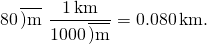Note that the unwanted meter unit cancels, leaving only the desired kilometer unit. You can use this method to convert between any type of unit. Now, the conversion of 80 m to kilometers is simply the use of a metric prefix, as we saw in the preceding section, so we can get the same answer just as easily by noting that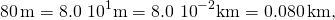since “kilo-” means 103 (see (Figure)) and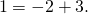However, using conversion factors is handy when converting between units that are not metric or when converting between derived units, as the following examples illustrate.

Example

Converting Nonmetric Units to Metric

The distance from the university to home is 10 mi and it usually takes 20 min to drive this distance. Calculate the average speed in meters per second (m/s). (Note: Average speed is distance traveled divided by time of travel.)

Strategy

First we calculate the average speed using the given units, then we can get the average speed into the desired units by picking the correct conversion factors and multiplying by them. The correct conversion factors are those that cancel the unwanted units and leave the desired units in their place. In this case, we want to convert miles to meters, so we need to know the fact that there are 1609 m in 1 mi. We also want to convert minutes to seconds, so we use the conversion of 60 s in 1 min.

Solution

1. Calculate average speed. Average speed is distance traveled divided by time of travel. (Take this definition as a given for now. Average speed and other motion concepts are covered in later chapters.) In equation form,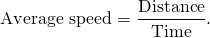2. Substitute the given values for distance and time: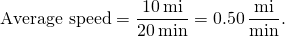3. Convert miles per minute to meters per second by multiplying by the conversion factor that cancels miles and leave meters, and also by the conversion factor that cancels minutes and leave seconds: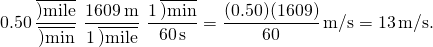Significance

Check the answer in the following ways:

1. Be sure the units in the unit conversion cancel correctly. If the unit conversion factor was written upside down, the units do not cancel correctly in the equation. We see the “miles” in the numerator in 0.50 mi/min cancels the “mile” in the denominator in the first conversion factor. Also, the “min” in the denominator in 0.50 mi/min cancels the “min” in the numerator in the second conversion factor.
2. Check that the units of the final answer are the desired units. The problem asked us to solve for average speed in units of meters per second and, after the cancellations, the only units left are a meter (m) in the numerator and a second (s) in the denominator, so we have indeed obtained these units.

Light travels about 9 Pm in a year. Given that a year is about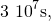what is the speed of light in meters per second?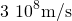Example

Converting between Metric Units

The density of iron is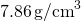under standard conditions. Convert this to kg/m3.

Strategy

We need to convert grams to kilograms and cubic centimeters to cubic meters. The conversion factors we need are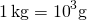and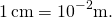However, we are dealing with cubic centimeters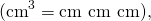so we have to use the second conversion factor three times (that is, we need to cube it). The idea is still to multiply by the conversion factors in such a way that they cancel the units we want to get rid of and introduce the units we want to keep.

Solution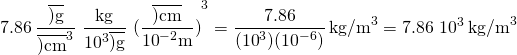Significance

Remember, it’s always important to check the answer.

1. Be sure to cancel the units in the unit conversion correctly. We see that the gram (“g”) in the numerator in 7.86 g/cm3 cancels the “g” in the denominator in the first conversion factor. Also, the three factors of “cm” in the denominator in 7.86 g/cm3 cancel with the three factors of “cm” in the numerator that we get by cubing the second conversion factor.
2. Check that the units of the final answer are the desired units. The problem asked for us to convert to kilograms per cubic meter. After the cancellations just described, we see the only units we have left are “kg” in the numerator and three factors of “m” in the denominator (that is, one factor of “m” cubed, or “m3”). Therefore, the units on the final answer are correct.

We know from (Figure) that the diameter of Earth is on the order of 107 m, so the order of magnitude of its surface area is 1014 m2. What is that in square kilometers (that is, km2)? (Try doing this both by converting 107 m to km and then squaring it and then by converting 1014 m2 directly to square kilometers. You should get the same answer both ways.)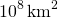Unit conversions may not seem very interesting, but not doing them can be costly. One famous example of this situation was seen with the Mars Climate Orbiter. This probe was launched by NASA on December 11, 1998. On September 23, 1999, while attempting to guide the probe into its planned orbit around Mars, NASA lost contact with it. Subsequent investigations showed a piece of software called SM_FORCES (or “small forces”) was recording thruster performance data in the English units of pound-seconds (lb-s). However, other pieces of software that used these values for course corrections expected them to be recorded in the SI units of newton-seconds (N-s), as dictated in the software interface protocols. This error caused the probe to follow a very different trajectory from what NASA thought it was following, which most likely caused the probe either to burn up in the Martian atmosphere or to shoot out into space. This failure to pay attention to unit conversions cost hundreds of millions of dollars, not to mention all the time invested by the scientists and engineers who worked on the project.

Given that 1 lb (pound) is 4.45 N, were the numbers being output by SM_FORCES too big or too small?

The numbers were too small, by a factor of 4.45.

Summary

• To convert a quantity from one unit to another, multiply by conversions factors in such a way that you cancel the units you want to get rid of and introduce the units you want to end up with.
• Be careful with areas and volumes. Units obey the rules of algebra so, for example, if a unit is squared we need two factors to cancel it.

Problems

The volume of Earth is on the order of 1021 m3. (a) What is this in cubic kilometers (km3)? (b) What is it in cubic miles (mi3)? (c) What is it in cubic centimeters (cm3)?

The speed limit on some interstate highways is roughly 100 km/h. (a) What is this in meters per second? (b) How many miles per hour is this?

a. 27.8 m/s; b. 62 mi/h

A car is traveling at a speed of 33 m/s. (a) What is its speed in kilometers per hour? (b) Is it exceeding the 90 km/h speed limit?

In SI units, speeds are measured in meters per second (m/s). But, depending on where you live, you’re probably more comfortable of thinking of speeds in terms of either kilometers per hour (km/h) or miles per hour (mi/h). In this problem, you will see that 1 m/s is roughly 4 km/h or 2 mi/h, which is handy to use when developing your physical intuition. More precisely, show that (a)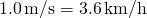and (b)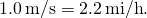a. 3.6 km/h; b. 2.2 mi/h

American football is played on a 100-yd-long field, excluding the end zones. How long is the field in meters? (Assume that 1 m = 3.281 ft.)

Soccer fields vary in size. A large soccer field is 115 m long and 85.0 m wide. What is its area in square feet? (Assume that 1 m = 3.281 ft.)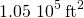What is the height in meters of a person who is 6 ft 1.0 in. tall?

Mount Everest, at 29,028 ft, is the tallest mountain on Earth. What is its height in kilometers? (Assume that 1 m = 3.281 ft.)

8.847 km

The speed of sound is measured to be 342 m/s on a certain day. What is this measurement in kilometers per hour?

Tectonic plates are large segments of Earth’s crust that move slowly. Suppose one such plate has an average speed of 4.0 cm/yr. (a) What distance does it move in 1.0 s at this speed? (b) What is its speed in kilometers per million years?

a.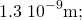b. 40 km/My

The average distance between Earth and the Sun is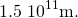(a) Calculate the average speed of Earth in its orbit (assumed to be circular) in meters per second. (b) What is this speed in miles per hour?

The density of nuclear matter is about 1018 kg/m3. Given that 1 mL is equal in volume to cm3, what is the density of nuclear matter in megagrams per microliter (that is,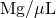)?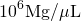The density of aluminum is 2.7 g/cm3. What is the density in kilograms per cubic meter?

A commonly used unit of mass in the English system is the pound-mass, abbreviated lbm, where 1 lbm = 0.454 kg. What is the density of water in pound-mass per cubic foot?

62.4 lbm/ft3

A furlong is 220 yd. A fortnight is 2 weeks. Convert a speed of one furlong per fortnight to millimeters per second.

It takes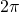radians (rad) to get around a circle, which is the same as 360°. How many radians are in 1°?

Light travels a distance of about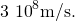A light-minute is the distance light travels in 1 min. If the Sun is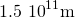from Earth, how far away is it in light-minutes?

A light-nanosecond is the distance light travels in 1 ns. Convert 1 ft to light-nanoseconds.

1 light-nanosecond

An electron has a mass of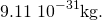A proton has a mass of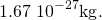What is the mass of a proton in electron-masses?

A fluid ounce is about 30 mL. What is the volume of a 12 fl-oz can of soda pop in cubic meters?